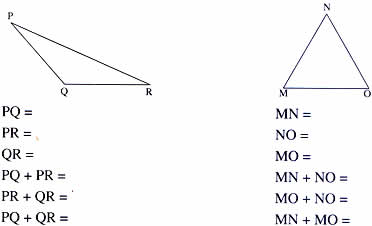Thursday , July 7 2022# NCERT 5th Class (CBSE) Mathematics: Polygons And Circles

B. In triangles ABC,

Side AB = 2 cm, BC = 3 cm, AC = 4 cm
AB + AC = 2 cm + 4 cm = 6 cm, AB + AC > BC
AB + BC = 5 cm, AB + BC > AC
AC + BC = 7 cm, AC + BC = AB

We can see that the sum of the lengths of any two sides of a triangle is more than the length of the third side. This is a property of all triangles.

Check this out for yourself.

Measure the sides of these triangles and compare the sum of the length of any two sides with the third side.CHECKPOINT

1. The sum of all three angles of a triangle is 180°.
2. The sum of the length of two sides of a triangle is always greater then the third side.

### Construction of Triangles

It is possibles to draw a triangle even if you do not have all the information about it.

You will need

1. a compass
2. a ruler
3. a protractor
4. a well sharpened pencil

## चुनौती हिमालय की 5th NCERT CBSE Hindi Rimjhim Ch 18

चुनौती हिमालय की 5th Class NCERT CBSE Hindi Book Rimjhim Chapter 18 प्रश्न: लद्दाख जम्मू-कश्मीर राज्य में …